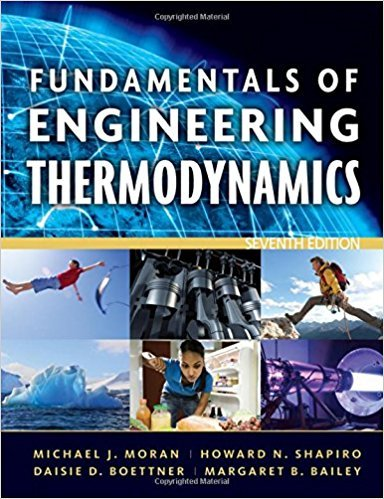×
Get Full Access to Fundamentals Of Engineering Thermodynamics - 7 Edition - Chapter 3 - Problem 57p
Get Full Access to Fundamentals Of Engineering Thermodynamics - 7 Edition - Chapter 3 - Problem 57p

×

# Solved: Applying the Energy BalanceA piston-cylinderISBN: 9780470495902 50

## Solution for problem 57P Chapter 3

Fundamentals of Engineering Thermodynamics | 7th Edition

• Textbook Solutions
• 2901 Step-by-step solutions solved by professors and subject experts
• Get 24/7 help from StudySoup virtual teaching assistantsFundamentals of Engineering Thermodynamics | 7th Edition

4 5 1 339 Reviews
30
2
Problem 57P

Problem 57P

Applying the Energy Balance

A piston-cylinder assembly contains water, initially saturated liquid at 150°C. The water is heated at constant temperature to saturated vapor.

(a) If the rate of heat transfer to the water is 2.28 kW, determine the rate at which work is done by the water on the piston, in kW.

(b) If in addition to the heat transfer rate given in part (a) the total mass of water is 0.1 kg, determine the time, in s, required to execute the process.

Step-by-Step Solution:

Solution 57P

Step 1 of 3:

In this question, we need to find heat transferred to water and determine the rate at which work is done by water on the piston inIn part b, we need to find the heat transferred in time inrequired to execute the process

Data given

TemperatureRate of heat transferMass of waterPart a:

Step 2 of 3

Step 3 of 3

##### ISBN: 9780470495902

Unlock Textbook Solution